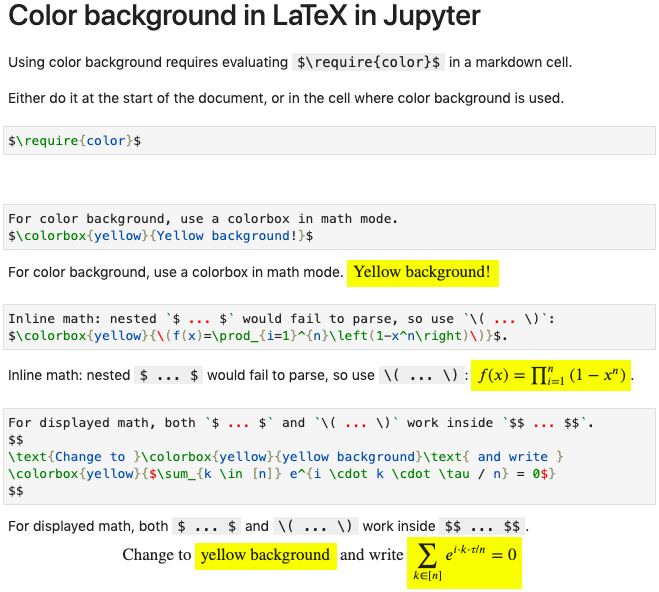# How change latex background text color in jupyter?

Expression Math( r"\color{green}{sample}") displays green text in latex in jupyter workbook. What is the directive to change the background color of the text? The directives \textcolor, \colorbox do not work.

edit retag close merge delete

Sort by » oldest newest most voted

Jupyter uses MathJax to render LaTeX. The reference for this question is therefore:

One solution indicated there involves modifying "the load array of the loader block of your MathJax configuration". I did not try that.

The other one, which I tried with success, consists in fist executing a markdown cell with

$\require{color}$


and then using \colorbox, for example:

$\colorbox{yellow}{Writing on yellow background!}$


This enables math fragments on color background, as part of displayed equations.

For instance:

$$\text{Change to }\colorbox{yellow}{yellow background}\text{ and write } \colorbox{yellow}{\sum_{k=1}^n e^{i \cdot k \cdot \tau / n} = 0}$$


For inline math, $\colorbox{yellow}{$ ... $}$ would not parse.

The trick is to use $\colorbox{yellow}{$...$}$ instead.

In the screenshot below, relevant markdown cells are duplicated, and showed once in edit mode and once in displayed mode.more

Thank you very much. This is it:

Math(r"\require{color}\colorbox{green}{a}")